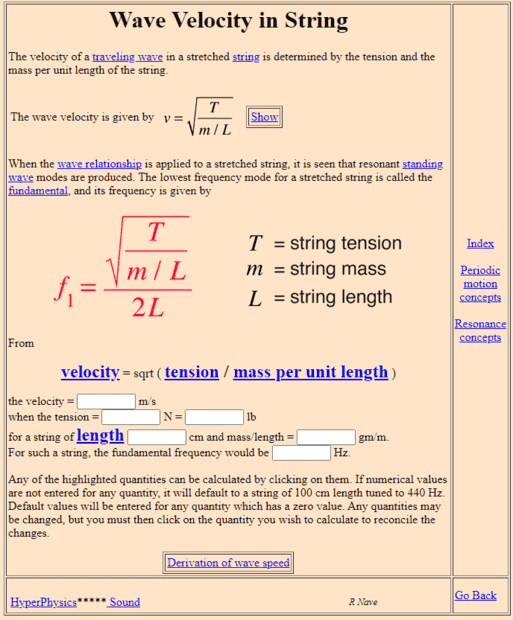# The speed of a waves on a string in Simple harmonic motion

• I
• annamal
The vertical displacement amplitude is assumed to be small in those derivations, so the tension in the string is not affected (in magnitude) by the wave passing by. Does that help?f

#### annamal

The speed of a wave in simple harmonic motion on a string is $$v= \sqrt{\frac{F}{\mu}}$$ where v= the horizontal velocity of the wave on a string.
Is the F the horizontal force or the resultant force (combination of Fy and Fx)?

The speed of a wave in simple harmonic motion on a string is $$v= \sqrt{\frac{F}{\mu}}$$ where v= the horizontal velocity of the wave on a string.
Is the F the horizontal force or the resultant force (combination of Fy and Fx)?
That "F" is the Tension force:http://hyperphysics.phy-astr.gsu.edu/hbase/Waves/string.html

••vanhees71, topsquark and dlgoff
Yes, I know that F is the tension but is F the tension of the string in the x direction or is F the tension that is tangent to the string.

My book derives it like this, implying that F is the tension of the string in the x directionThe speed of a wave in simple harmonic motion on a string is $$v= \sqrt{\frac{F}{\mu}}$$ where v= the horizontal velocity of the wave on a string.
Is the F the horizontal force or the resultant force (combination of Fy and Fx)?
It does not matter. To the approximation that is used to derive the wave equation, the difference is negligible.

•vanhees71 and topsquark
•topsquark and annamal
Yes, this makes the tension of the string in the horizontal direction as well

It does not matter. To the approximation that is used to derive the wave equation, the difference is negligible.
Ok, not sure how the tension in the x direction can be approximated as the resultant tension

Ok, not sure how the tension in the x direction can be approximated as the resultant tension
The vertical displacement amplitude is assumed to be small in those derivations, so the tension in the string is not affected (in magnitude) by the wave passing by. Does that help?

•topsquark and annamal
For a typical steel string guitar a 2mm displacement at the midway point translates into an increase in string tension on the order of one part in one thousand. So it's a pretty good approximation to just assume that the tension doesn't change. Then you can focus on the vertical force and come up with a straightforward equation for the speed of the wave.